Next: 4.3 Angular Size Up: 4. Statistical Model of Previous: 4.1 Morphological Classification   Contents

# 4.2 Number Counts

Galaxy differential number counts, giving the number of galaxies per unit sky area per unit magnitude interval as function of total magnitude, have always been a classical tool of observational cosmology. Consequently, a great effort has always been devoted to the extension of the observations to deeper magnitudes, larger sky regions and a wider range of colors. In particular, in the past few years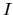-band counts at high Galactic latitudes have been reliably extended down to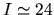, as summarized e.g. by [Shimasaku and Fukugita 1998]. In our model, counts from three different sources were combined in order to cover as large a magnitude range as possible. At bright magnitudes, i.e. for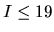, well-established counts were provided by [Lattanzi 1997], whereas at fainter magnitudes results from [Glazebrook et al. 1995] (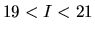) and [Abraham et al. 1996b] (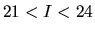) were used. A least-square polynomial fit in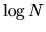vs.was performed on these data, in order to assess the consistency of the three sources and to obtain a functional form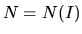for use in the following. It was thus found that a second degree polynomial was sufficient to obtain a good fit to the data. The number counts and the best-fit parabola are shown in Figure 4.2, while the best-fit parameters are given in Table 4.2. According to this approximation, the differential number counts take the following functional form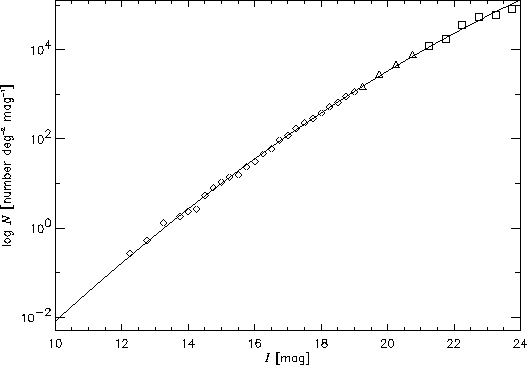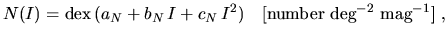(4.1)

where dex'' stands for the exponential function in base ten.

Values of the three parameters contained in Equation 4.1 are given in Table 4.2, while counts calculated with this formula are given in Table 4.4.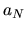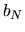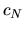-9.9942 0.90564 -0.011493

The cumulative galaxy number counts, giving the total number of galaxies per unit sky area brighter than a givenmagnitude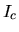, are then given by the definite integral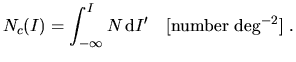(4.2)

Since the function given by Equation 4.1 does not have an analytic antiderivative, Romberg numerical integration (see Chapter 4 in [Press et al. 1996]), was performed. Cumulative galaxy number counts that were thus obtained are listed in Table 4.4.Next: 4.3 Angular Size Up: 4. Statistical Model of Previous: 4.1 Morphological Classification   Contents
Mattia Vaccari 2000-12-05